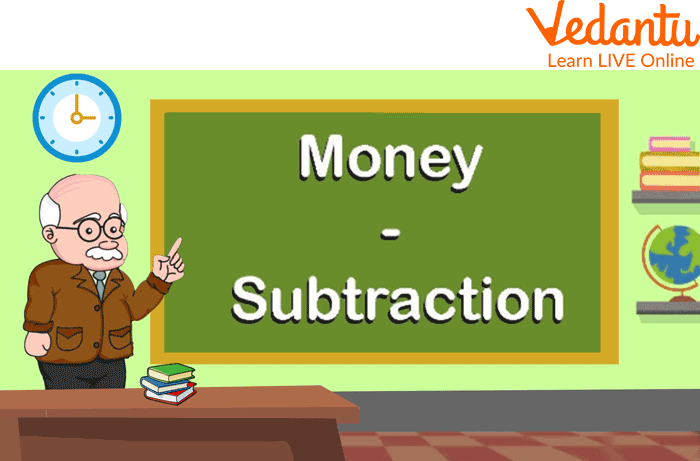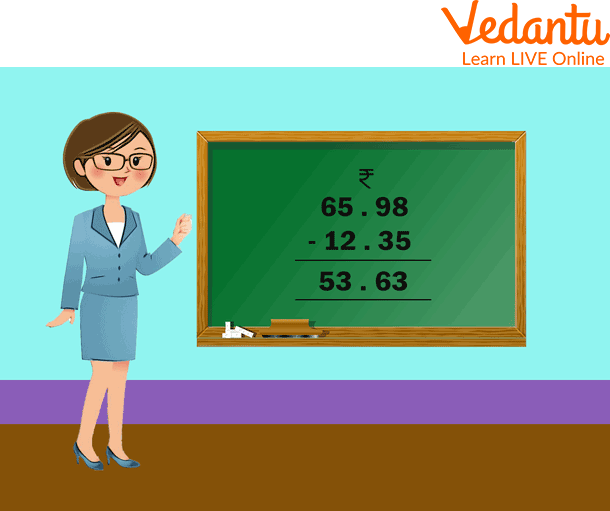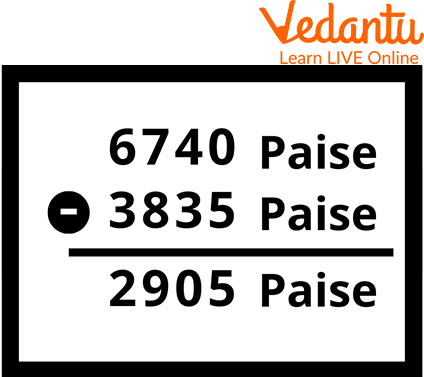Courses
Courses for Kids
Free study material
Free LIVE classes
More

# Subtraction of Rupees and PaiseLIVE
Join Vedantu’s FREE Mastercalss

## Introduction to Subtraction of Money

The subtraction operation is the most popular arithmetic operation among children. The kids subtract rupees and paise from whatever they have. Rupees and paise are two different measurements of Indian money. They are often mixed up, and people might think paise is the same as rupees, but they're not. This article will teach you how to differentiate between the terms rupee and paisa; also, it includes the meaning, methods, and steps of subtraction of money.Subtraction of Money

## Subtraction Methods Based on Rupees and Paise

We will learn how to subtract money values that include rupees and paise in order to get the difference. Money is subtracted in the same way as decimal numbers are subtracted. We must ensure that the sums of money are changed into paisa while subtracting. We will discover two distinct approaches to resolving subtraction problems with rupees and paise. Students can use both strategies.

• Subtracting the values and changing them to rupees.

• Subtracting the amounts without changing them to rupees.Subtraction of Money

## Conversion of Rupees and Paise

Below is the example for the addition and subtraction of rupees and paise conversion:

• Paise to Rupees Conversion: While converting paise into rupees, we divide paise by 100. Converting paise into rupee formula is to divide the paise by a hundred.

Example 1. Convert 800 paise into rupees.

Ans: Here, we have 800 p

For converting, we will divide it by 100.

=\$\dfrac{800}{100}\$ = ₹ 8.00

Therefore, by converting 800 paise into rupees, we get 8 rupees.

• Rupees to Paise Currency Conversion: Rupees conversion into paise happens when we multiply the rupees by a hundred.

Example 2. Convert ₹ 4 into paise.

Ans: ₹ 4 = 4 × 100 = 400 p

Therefore, ₹ 4 will give 400 p on conversion.

## Benefits of Money Subtraction

• The children will learn about common situations involving money through these math worksheets and experience dealing with subtraction involving different currencies.

• The subtracting money worksheets are purposefully made with an emphasis on how to handle financial transactions, find prices, figure out how much change to give and how much tax to pay, as well as receive adequate practice with mental Maths.

## Solved Examples

1. Subtract Rs. 38.55 from Rs. 67.40

Solution:

Method - with conversion into paise:

Rs. 67.40 = 6700 paise + 40 paise = 6740 paise

Rs. 38.35 = 3800 paise + 35 paise = 3835 paiseSolved Example

Therefore, difference of Rs. 38.35 from Rs. 67.40 = 2905 Paise = Rs. 29.05

2. Add Rs. 35.25 and Rs. 125.75

Ans: As we know that we write rupee and paise by separating them with a dot.

In the example of adding 35.25 and 125.75, firstly we write them in vertical addition form.

We add the paise first and then the rupees.

The final answer to this question would be 161.00 Rupees.

## Subtraction of Rupees and Paise Worksheet

(i) Rs. 236. 00 – Rs. 43.00 (Ans: Rs. 236. 00 – Rs. 43.00 = Rs. 193)

(ii) Rs. 546.00 – Rs. 318.00 (Ans: Rs. 546.00 – Rs. 318.00= Rs. 228)

(iii) 528.00 p – 207.00 p (Ans: 528.00 p – 207.00 p = 321 p)

(iv) Rs. 587.00 – Rs. 419.00 (Ans: Rs. 587.00 – Rs. 419.00 =Rs. 178)

(v) Rs. 656.00 – Rs. 145.00 (Ans: Rs. 656.00 – Rs. 145.00 =Rs. 511)

## Summary

We deal with rupees and paise in our daily lives. Nothing can be bought without money. Money is required to do everything. In ancient times, paise was also of use, but now only rupees are in use. The formulas to convert the rupee into paisa and paise into rupee are to be understood well. To convert rupees into paisa, we just have to multiply by 100.

Similarly, to convert paisa to rupees we just have to divide by 100. These concepts are combined with multiplication and division as well. Children must know how to deal with money. These concepts have to be taught well to the kids.

Last updated date: 15th Sep 2023
Total views: 97.8k
Views today: 0.97k

## FAQs on Subtraction of Rupees and Paise

1. How many 50 paise coins will I get in rupees?

We know that 1 Rupee is equal to 100 paise, so we can now say that two 50 paise coins make 1 rupee.